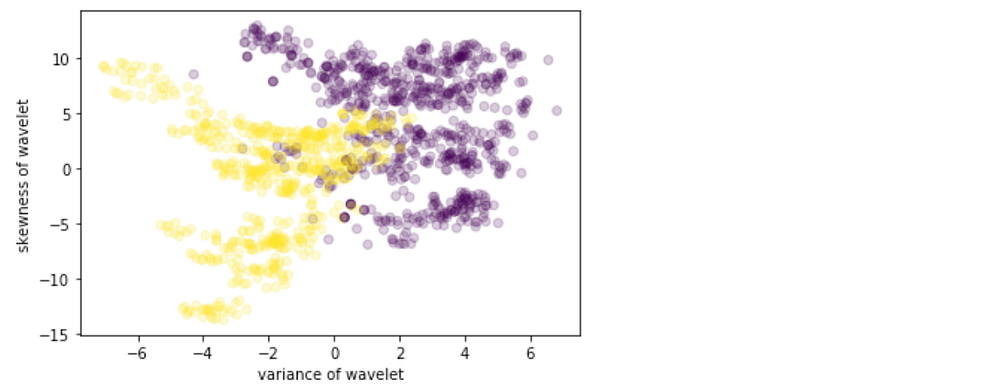# Building a Neural Network with a Single Hidden Layer using Numpy

Original article was published on Deep Learning on Medium# Building a Neural Network with a Single Hidden Layer using Numpy

Implement a 2-class classification neural network with a single hidden layer using Numpy

In the previous post, we discussed how to make a simple neural network using NumPy. In this post, we will talk about how to make a deep neural network with a hidden layer.

1. Import Libraries

We will import some basic python libraries like numpy, matplotlib (for plotting graphs), sklearn (for data mining and analysis tool), etc. that we will need.

`import numpy as npimport matplotlib.pyplot as pltfrom sklearn.model_selection import train_test_split`

2. Dataset

We will use the Banknote Dataset that involves predicting whether a given banknote is authentic given several measures taken from a photograph. It is a binary (2-class) classification problem. There are 1,372 observations with 4 input variables and 1 output variable. For more detail see the link.

`data = np.genfromtxt(‘data_banknote_authentication.txt’, delimiter = ‘,’)X = data[:,:4]y = data[:, 4]`

We can visualize the dataset using a scatter plot. We can see two classes (authentic and not authentic) are separable. Our goal is to build a model to fit this data i.e. we want to build a neural network model that defines regions as either authentic or unauthentic.

`plt.scatter(X[:, 0], X[:, 1], alpha=0.2, c=y, cmap=’viridis’)plt.xlabel(‘variance of wavelet’)plt.ylabel(‘skewness of wavelet’);`